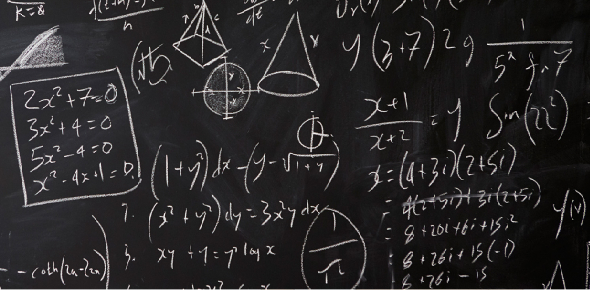# M - Operations With Integers

10 Questions | Total Attempts: 24SettingsStudents will demonstrate their proficiency with adding, subtracting, multiplying, and dividing integers.

Related Topics
• 1.
Give an example of a subtraction problem using one positive integer and one negative integer and solve it correctly.
• 2.
-3 + (-5) =
• A.

2

• B.

-8

• C.

8

• 3.
-19 + 5 =
• A.

-24

• B.

24

• C.

-14

• 4.
-8 - 8 =
• A.

-16

• B.

0

• C.

16

• 5.
12 - (-7) =
• A.

5

• B.

-19

• C.

19

• 6.
-4 - (-3) =
• A.

-1

• B.

7

• C.

1

• 7.
5 x (-2) =
• A.

10

• B.

-10

• C.

0

• 8.
-8 x (-3) =
• A.

32

• B.

-32

• C.

24

• 9.
The rule for multiplying and dividing integers is to look at the signs of the integers in the problem, if the signs are the same (like signs) you keep the sign, if the signs are different, you keep the sign of the larger absolute value.
• A.

True

• B.

False

• 10.
The rule for adding integers is to look at the signs of the integers in the problem, if the signs are the same (like signs) you add and keep the sign, if the signs are different, you subtract the absolute values of the integers and keep the sign of the larger absolute value.
• A.

True

• B.

False